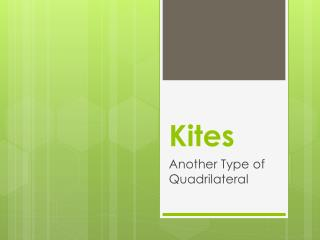# Kites - PowerPoint PPT PresentationDownload PresentationKites

KitesDownload Presentation## Kites

- - - - - - - - - - - - - - - - - - - - - - - - - - - E N D - - - - - - - - - - - - - - - - - - - - - - - - - - -
##### Presentation Transcript

1. Kites Another Type of Quadrilateral

3. Trapezoids and Kites • A Trapezoid is a quadrilateral because it has four sides. It isNOT a parallelogram because both pairs of opposite sides are not parallel. • Another type of quadrilateral that isNOTa parallelogram is a Kite. • Definition of a Kite: • Kite– A quadrilateral with two pairs of congruent, adjacent sides.

4. Properties of a Kite • The diagonals of a kite are perpendicular. The longer diagonal is the bisector of the shorter diagonal. • There is exactly one pair of opposite angles that are congruent. • There are two consecutive pairs of congruent sides.

5. Examples of Kite Properties

6. Example #1 • Given: GJIH is a Kite. HG=HI and IJ=GJ. The following questions are true or false. True False True False True False

7. Example #2 • Given: GJIH is a Kite. HI = 10, GJ = 18. IK = 8 and KG = 8. Find the following values: • HG = • IJ = • IG = • HK = • KJ = • HJ = 10 18 16 6

8. Example #3 • Given: MLRN is a Kite. Given, ML = 10, LR = 17, LA = 8, AML = 53, MLK = 99. Find the following values: • NR = • Perimeter of MLRN = • IN = • ALR = • MLA = • MNR =

9. Example #4 • Given: WXYZ is a Kite. WX = YX and WZ=YZ. Find the following angles: • VYZ = • VXY = • WZY = • XWZ = • WXY =